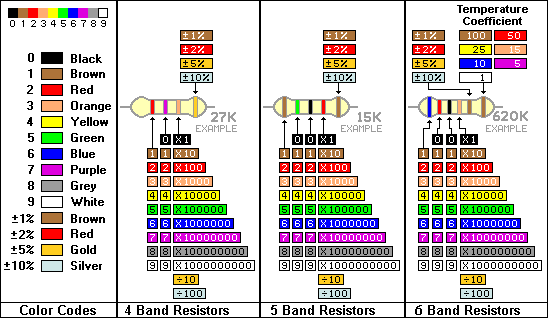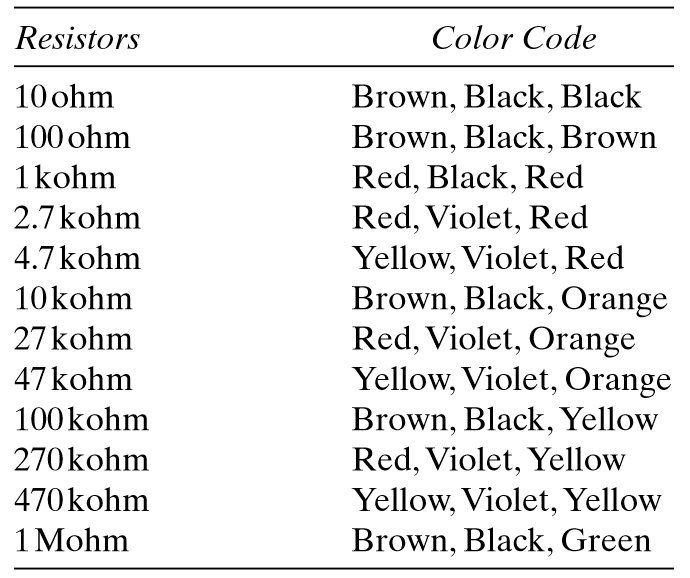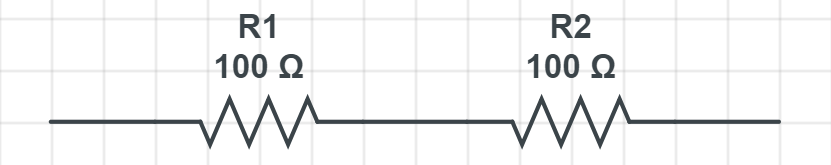# Everything about Resistors

Resistors are not only the most common type of component found in electronics construction projects, but they are also the simplest to use. A resistor can be recognized as a small, tubular-shaped component with a wire lead coming from each end and a series of color bands on the body. Electrical resistance is measured in units of ohm, with higher values being preceded by the word kilo for thousands of ohm, thus 10 kohm is equivalent to 10,000 ohm.

## Resistor Color Code

A resistor’s resistance value is deciphered with the color code shown as follows. Each color corresponds to a particular number. It is advisable to take the time to learn the color code.Basically, three color bands are used to represent the resistor value. For example, a 100-ohm resistor has the color bands brown, black, brown. The ﬁrst band color (brown) has a value of 1, the second band color (black) has a value of 0, and the last color (brown) has a value of 1.

The ﬁrst two colors always represent the ﬁrst and second numbers of the resistor value, and the third color indicates the number of zeros needed after the second number. Thus we have brown, black, brown = 100 ohms.

By going through a few more examples, you will soon see the pattern emerging.A 27-Kohm resistor is represented by the color code red,violet,orange = 27,000ohm = 27kohm

47-Kohm resistor has the color bands yellow,violet,orange = 47,000ohm = 47kohm

One thousand ohms is represented by the letter k; that is, 1,000ohm is the same as 1kohm, and 27,000 ohm is the same as 27kohm. A value such as 2,700ohm is, therefore, the same as 2.7kohm.

For even larger values, the letter M represents one million ohms. Thus, 1,000,000 equals one million ohm or 1 Mohm. In dealing with resistors for construction projects, it is necessary to know the color code in order to select the correct resistor value.

Let A,B,C and D are color bands of resistor, then the Resistance R is given by

R=AB x 10^C (+ or -) D

## Resistor Tolerance

You should be familiar with translating color codes to resistor values and, conversely, resistor values to color codes. At this stage, we need to introduce a fourth color band. This band, typically either gold or silver represents the tolerance of the resistor, gold being a 5-percent tolerance resistor and silver a 10-percent tolerance resistor.

Tolerance relates to the allowable spread in resistance value. Assume that we have a 100-ohm resistor, so the color bands are brown, black, brown, with a fourth gold (5 percent) band. This means that the actual resistance value could be 100ohm plus 5 percent or 100ohm minus 5 percent. Five percent of 100ohm is 5ohm.

Therefore, this resistor marked with a 100-ohm value could, in reality, be between 100ohm plus 5ohm or 100ohm minus 5ohm; that is, between 105ohm and 95ohm.

The resistor should be oriented so that the gold or silver band is at the right-hand side and the resistance value is then read from left to right. Instead of the gold (5 percent) or silver (10 percent) band, resistors can also have no color to represent a 20-percent tolerance.

## Resistor Power Rating

Another feature of the resistor is its power rating. Typically, a small 1/4watt-size resistor is most commonly used, especially for the projects described in this book. The physical size of the resistor gives you an indication of its power rating. If resistors are bought several to a packet, then the power rating will be marked on the packet.

The next larger power rating, the 1/2-watt resistor, can be used as a substitute for the 1/4-watt resistor but is physically larger and, therefore, takes up more board space. This is a waste because the smaller, more compact 1/4-watt resistor is used in most cases. One of the common uses for a resistor is to limit the current ﬂowing through a circuit.

A low-value resistor allows more current to ﬂow and a high-value resistor allows less current to ﬂow. A good example of this property would be the case of a battery supplying current to a ﬂashlight bulb. By placing a resistor between the battery and lightbulb, the current would be reduced and the light would be dimmed.

A wide range of resistors are available between 1ohm and 1Mohm. Practically, however, I ﬁnd that the following range is comfortably smaller and hence more manageable. You might want to consider using this as a starter range when buying components.Over the years, these 12 values have covered more than 90 percent of my project resistor needs, all in the 1/4-watt power rating value.

Resistors are robust and unlikely to be damaged. They have the added advantage over other electronic components as being the simplest to use in electronic circuits. When bending resistor leads (for soldering purposes as explained later), always make sure that the wire is not bent ﬂush to the resistor body. Leave a small gap (about 1/16 inch is ﬁne) so the resistor body does not fracture with the bend.

## Resistor Series Connection

Resistors can be increased in value by the simple method of connecting them in a series, that is to say, one lead of the ﬁrst resistor is connected to the second resistor. The increased value is measured across the two free ends. For example, two resistors with individual values of 10kohm would add up to 20kohm when connected in a series. This is a useful tip when you need a speciﬁc resistor value that is larger than you might have on hand. The ﬁnal resistor value can be calculated from

Rtotal = R1 + R2## Resistor Parallel Connection

Another way of connecting resistors is in parallel. In this case, one end of the ﬁrst resistor goes to one end of the second resistor, and the two free ends are also connected together. The total resistance this time is a little more complicated to calculate and is given by

1/Rtotal = 1/R1 + 1/R2For example, if the two resistors were equal in value, say 10kohm, then the total parallel resistance would be 5kohm. If the two resistors were unequal in value, then the total parallel combination is always less the smaller of the two resistors. For example, a 4.7-Kohm and 10-Kohm resistor in parallel results in a 3.2-Kohm resistor. This is another tip for creating a smaller resistor value than you might have.